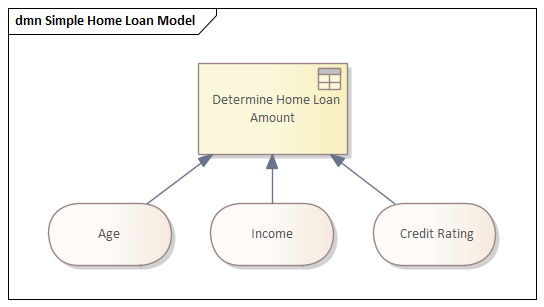﻿ Modeling with DMN | Enterprise Architect User Guide
 Prev Next

Modeling with DMN

This topic introduces you to the most important elements that you need to create Decision models. As discussed in earlier topics there are two fundamental parts of a decision model namely the Decision Requirements diagram and the decision logic. Creating a decision diagram is straight forward and probably the most onerous part of the exercise will be unraveling the way an organization makes decisions and what the inputs to these decision are. The diagrams will typically contain decisions that are chained together describing the fact that one decision can provide input to another decision and so on.Simple Decision Requirements diagram showing three inputs into a Decision using a Decision Table.

The logic of the decision is described using a number of devices but the most commonly used and accessible form is the Decision Table. The Decision Table contains rows and columns like a spreadsheet and covers all the possible combinations of inputs to produce a number of outputs. For example if an applicant is older than 21 and younger than 65 and earns \$60,000 per year with a good credit rating the bank will lend them \$300,000 for a home loan.

A Decision Table showing three inputs and one output, rows would be added to define the rules.